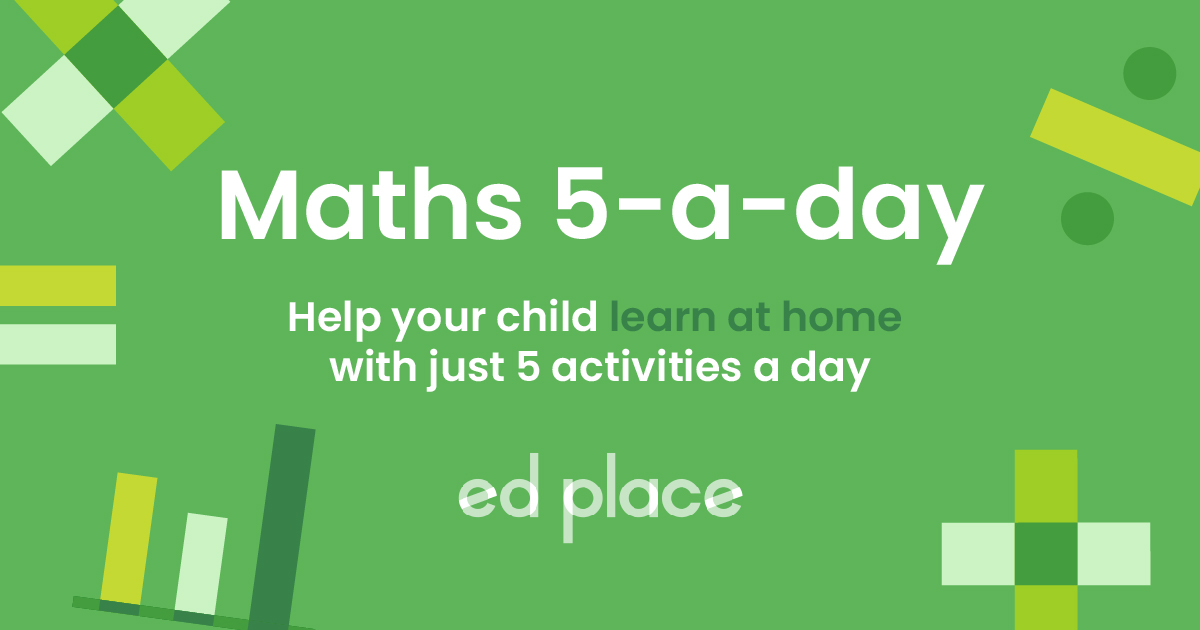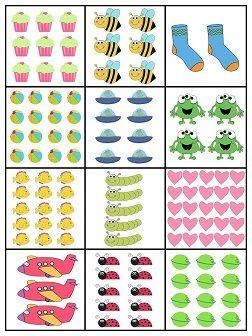# EdPlace's KS1 home learning maths lesson: Solving Word Problems in Maths

Looking for short lessons to keep your child engaged and learning? Our experienced team of teachers have created English, maths and science lessons for the home, so your child can learn no matter where they are.  And, as all activities are self-marked, you really can encourage your child to be an independent learner.

Get them started on the lesson below and then jump into our teacher-created activities to practice what they've learnt. We've recommended five to ensure they feel secure in their knowledge - 5-a-day helps keeps the learning loss at bay (or so we think!).

Are they keen to start practising straight away? Head to the bottom of the page to find the activities.

Now...onto the lesson!

Key Stage 1 Statutory Requirements for Maths
Year 1 students should be able to solve one-step problems that involve addition, subtraction, multiplication and division, using concrete objects and pictorial representations, and missing number problems such as 7 = – 9.
Year 2  students should be able to solve problems involving multiplication and division, using materials, arrays, repeated addition, mental methods, and multiplication and division facts, including problems in contexts.

## What should our KS1 mathemagicians know?

Word problems pop up frequently in Maths lessons and are designed to see whether a child can apply their knowledge of different mathematical concepts to various ‘real life’ situations. Word problems are a key part of the ‘reasoning’ aspect of the Maths curriculum and show a child has grasped a deep understanding of a subject.

We believe that if you follow our step by step approach your child will:

1) Understand how to follow some simple steps to solve a worded problem

2) Describe the calculation needed to crack the problem

3) Explain the process that they used to you at the end

## Step 1 - Language check!

Before we get going, it's crucial to check your child understands the important language we will use for this lesson.  Addition is the method of adding two or more numbers together to find a total. Think of adding as ‘putting together’.  Subtraction is the ‘taking away’ of one number from another, to make the original number smaller. Think of subtracting as 'taking apart'.  Multiplication is an operation in which a number is added to itself several times.  Division simply means ‘sharing equally' into groups. Using real-life scenarios is a helpful way for children to grasp division, e.g. the sharing of toys or sweets.

## Step 2 - Key Words, Plus a Bit More Context...

To tackle this lesson, your child will need a sound understanding of these key concepts.

Addition: Adding two or more given numbers together to find a total, we can call this the total sum of the numbers. Initially, your child will have been taught to add using concrete objects and will then have progressed onto using a number line.

Subtraction: Subtracting allows us to find the difference between two values. Remember to subtract the smallest number from the largest. Again, your child will have been taught to subtract using concrete objects and will then have progressed onto using a number line to count backwards.

Multiplication: Your child will so far have been taught multiplication as ‘repeated addition’ using a number line, they may also draw arrays (drawing dots in rows) to multiply. Remind your child that multiplication can be done in any order.

For example, look at the bees in the picture below.  We can see that this array shows 2 x 3 or 3 x 2, and we get the same answer of 6, whichever way we do it!Division: Your child should have been taught to divide with real objects by 2, 5 and 10. By the end of Year 2, children should be fluent in the 2, 5 and 10 x table, so it makes sense to divide by these numbers too. You could practice at home by giving them 10 pennies and asking them to divide by 2 or 5.

To solve a worded problem, children need a logical way to approach it. A child can be faced with a worded problem in maths and forget all the fantastic maths they've learned because they are so overwhelmed to have some words thrown into the question! Remember, to encourage your child to ask for help if they are finding it difficult to read the question, it can often help them to hear an adult read it aloud.

Luckily, there is a commonly used acronym that can help your child to feel confident to solve a worded problem… we like to use the word RUCSAC

Understand - Do you understand what the problem is?

Choose - Can you choose whether you’ll need to use a ÷ × - or a  +

Solve - Can you pull out the sum from the problem and work it out?

Answer - Can you write the answer? Check that you’ve answered the question, what did you need to find out in the first place?

Check - Can you check that you got the answer right?

## Step 4 - Putting it Altogether!

Now, have a go using the acronym RUCSAC to solve these worded problems together. Remember that in both Year 1 and 2, a child would usually be given some apparatus like a number line to help them solve a worded problem.

1. Sarah had 28 coins in her piggy bank. Her Dad gives her 4 more coins as pocket money. How many coins does she have now?

2. A box has 6 eggs inside. How many eggs will there be in 3 boxes?

3. Zach made 12 biscuits. Half of the biscuits were eaten, how many are left?

4. Ethan bought 5 books about animals, 9 books about outer space, and 3 books about trains. Each book cost £10. How much did Ethan spend on the books?

## Step 5 - Give it a go...

We all know that practice makes perfect, so why not assign your child the following five activities to test what they've learned?

All activities are created by teachers and automatically marked. Plus, with an EdPlace subscription, we can automatically progress your child at a level that's right for them. Sending you progress reports along the way so you can track and measure progress, together - brilliant!

a) 28 + 4 = 32 coins

b) 6 x 3 = 18 eggs

c) 12 ÷ 2 = 6 biscuits

d) Step 1: find the total number of books:  5 + 9 + 3 = 17

Step 2: find the cost of the books: £10 x 17 = £170

£170 spent on the books.

### Keep going! Looking for more activities, different subjects or year groups?

Click the button below to view the EdPlace English, maths, science and 11+ activity library